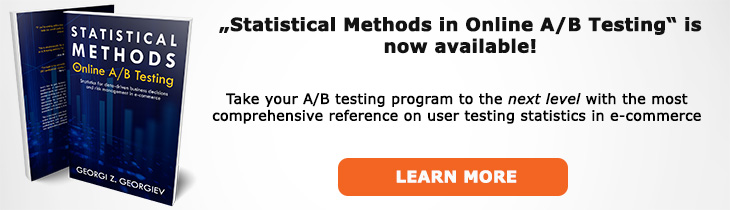# What does "Sufficient Estimator" mean?

Definition of Sufficient Estimator in the context of A/B testing (online controlled experiments).

## What is Sufficient Estimator?

An estimator of a parameter θ which gives as much information about θ as is possible from the sample at hand is called a sufficient estimator. Sufficient estimators exist when one can reduce the dimensionality of the observed data without loss of information. In A/B testing the most commonly used sufficient estimator (of the population mean) is the sample mean (proportion in the case of a binomial metric). A conversion rate of any kind is an example of a sufficient estimator.

In a more formal expression it can be said that a statistic is sufficient with respect to an unknown parameter and a given family of probability distributions if the sample from which it is calculated gives no additional information as to which of those probability distributions produced it than does the statistic itself. Thus sufficiency refers to how well an estimator utilizes the information in the sample relative to the postulated statistical model.

Sufficiency is an important quality in hypothesis testing where we are effectively comparing the distribution under the null hypothesis with the actually observed distribution. Having a sufficient estimator makes this process significantly more manageable, especially for large sample sizes.

Like this glossary entry? For an in-depth and comprehensive reading on A/B testing stats, check out the book "Statistical Methods in Online A/B Testing" by the author of this glossary, Georgi Georgiev.## Glossary Index by Letter

Select a letter to see all A/B testing terms starting with that letter or visit the Glossary homepage to see all.